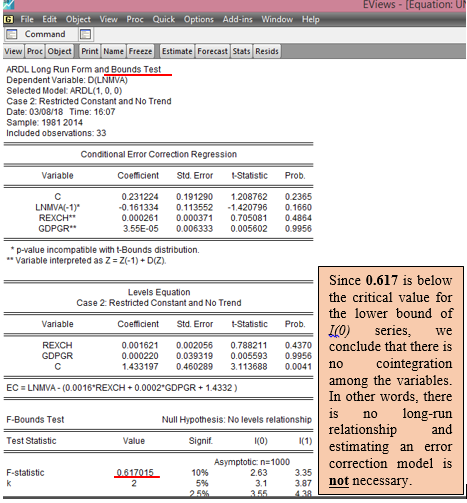## After unit root testing, what next?

In the Part 1 of this structured tutorials, we discussed Scenario 1: when the series are stationary in levels that is I(0) series and Scenario 2: when they are stationary at first difference. In the first scenario, it implies that any shock to the system in the short run quickly adjusts to the long run. Hence, only the long run model should be estimated.  While for the second scenario, the relevance of the variables in the model is required, therefore there is need to test for cointegration. If there is cointegration, specify the long-run model and estimate VECM but if otherwise, specify only the short-run model and apply the VAR estimation technique and not VECM. In today’s lecture we consider the third scenario of when the variables are integrated of different orders.

Scenario 3: The series are integrated of different orders?
1.    Should in case the series are integrated of different orders, like the second scenario, cointegration test is also required but the use of Johansen cointegration test is no longer valid.
2.    The appropriate cointegration test is the Bounds test for cointegration proposed by Pesaran, Shin and Smith (2001)
3. The estimation technique to apply is not VAR but the autoregressive distributed lag (ARDL) model.
4.  Similar to scenario 2, if series are not cointegrated based on Bounds test, we are expected to estimate only the short run. That is, run only the ARDL model (where variables are neither lagged nor differenced). It is the static form of the model.
5.    However, both the long run and short run models are valid if there is cointegration. That is, run both ARDL and ECM models.

Bounds Cointegration Test in EViews
In this example, we use the Dar.xlsx data on Nigeria from 1981 to 2014 and the variables are the log of manufacturing value-added (lnmva), real exchange rate (rexch) and gross domestic growth rate (gdpgr). The model examines the effect of real exchange rate on manufacturing sector while controlling for economic growth.

Note: Cointegration test should be performed on the level form of the variables and not on their first difference. It is okay to also use the log-transformation of the raw variables, as I have done in this example.

Step 1: Load data into EViews (see video on how to do this)
Step 2: Open variables as a Group data (see video on how to do this) and save under a new name
Step 3: Go to Quick >> Estimate Equation >> and specify the static form of the model which is stated as: lnmvat = b0 + b1rexcht + b2gdpgrt + ut in the Equation Estimation WindowEViews Estimation Equation Dialog BoxSource: CrunchEconometrix

Step 4: Choose the appropriate estimation technique
Click on the drop-down button in front of Method under Estimation settings and select ARDL – Auto regressive Distributed Lag Models

Step 5: Choose the appropriate maximum lags and trend specification
The lag length must be selected such that the degrees of freedom (defined as n - k) must not be less than 30. The Constant option under the Trend specification is also selected.

Step 6: Choose the appropriate lag selection criterion for optimal lag
Click on Options tab, then click on the drop-down button under Model Selection Criteria and select the Akaike info Criterion (AIC), then click Ok.EViews: Information Criterion SelectionSource: CrunchEconometrix
Step 7: Estimate the model based on Steps 3 to 6EViews: ARDL OutputSource: CrunchEconometerix

Step 8: Evaluate the preferred model and conduct Bounds test
The hypothesis is stated as:
H0: no cointegrating equation
H1: H0 is not true
Rejection of the null hypothesis is at the relevant statistical level, 10%, 5% level, 1%.

a. Click on View on the Menu Bar
b. Click on Coefficient Diagnostics
c. Select the Bounds Test option

The following result is displayed below:
Here is the EViews result on the ARDL Bounds Test of lnmva, rexch and gdpgr:EViews: ARDL Bounds Test ResultSource: CrunchEconometrix

Step 9: Interpret your result appropriately using the following decision criteria
The three options of the decision criteria are as follows:
1.    If the calculated F-statistic is greater than the critical value for the upper bound I(1), then we can conclude that there is cointegration that is there is long-run relationship.
2.    If the calculated F-statistic falls below the critical value for the lower bound I(0) bound, then we conclude that there is no cointegration, hence, no long-run relationship
3.    The test is considered inconclusive if the F-statistic falls between the lower bound I(0) and the upper bound I(1).

Decision: The obtained F-statistic of 0.6170 falls below the lower bound I(0), hence, we will consider only short run models since the variables show no evidence of a long-run relationship as indicated by the results from the Bounds test.

[Watch video on how to conduct Bounds test for cointegration in EViews]

If there are comments or areas requiring further clarification, kindly post them below….